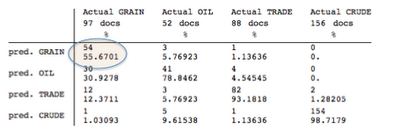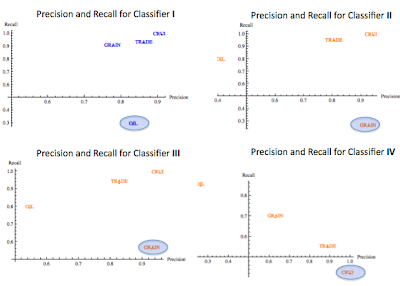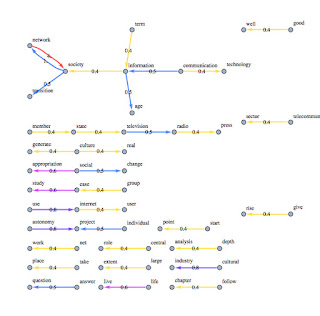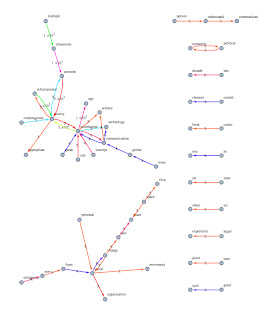Monday, January 30, 2012

Document Classification: how to boost your classifier

Time ago, we discussed about ADAboost as a meta algorithm useful to improve the accuracy of a weak classifier and I showed a sample on a boolean classification problem.
Frund and Schapire in this excellent paper proposed a version of this algorithm adapted to work with "multi labels" classifiers.

The two variants proposed in such paper are based on the trick to assign a weight to each sample of the training set in according with the error committed by the classifier, so it forces the classifier to be focused on the hard instances of the problem.
The improved classifier is finally obtained as a weighted combination of each version of the weak algorithm improved at each iteration.

ADaBoost.M1 tries to improve step by step the accuracy of the classifier analyzing its behavior on training set. (Of course you cannot try to improve the classifier working with the test set!!).
Here lays the problem, because if we choose as "weak algorithm" an SVM, we know that almost always it returns excellent accuracy on the training set with results closed to 100% (in term of true positive).
In this scenario, try to improve the accuracy of classifier assigning different weights to the training set doesn't work!

The idea of AdaBoost.M1 is still valid also with SVM classifiers, but I noticed that instead of to improve step by step the single SVM classifier,  we can train different SVM classifiers (with different parameters) and assign the score to each classifier based on the error committed on a part of the training set not used to train the SVM.
Let me explain better the procedure:

1. Split the training set in two different sets: training set (80% of the volume of the original training set), boosting set (20% of the volume of the original training set).
2. train k SVM classifiers with different parameters.
3. test the above SVM on the boosting set and calculate the beta score as done in the original implementation of ADAboost.M1.
4. The final answer of the boosted classifier is obtained as proposed by the original implementation of the Adaboost.M1

As you can notice, the method basically assigns to the k classifiers a "trustability score".

Real Case
Let's see what happens with our REUTERS-2158 data set:
I tried to classify the documents belonging to the categories: "GRAIN","CRUDE", "TRADE","OIL".
I trained four different SVM. Here you are what are the results of each classifier:The Confusion Matrix for the Classifier I.The principal diagonal represents the True Positive. In The circle the worst result for this classifier.The Confusion Matrix for the Classifier II.The principal diagonal represents the True Positive. In The circle the worst result for this classifier.The Confusion Matrix for the Classifier III.The principal diagonal represents the True Positive. In The circle the worst result for this classifier.The Confusion Matrix for the Classifier IV.The principal diagonal represents the True Positive. In The circle the worst result for this classifier.
And after the application of the meta algorithm, here you are the confusion matrix for the improved classifier:The Confusion Matrix for the Boosted classifier
As you can see the boosting provides us really good results!
Here the same results showed in terms of precision and recall:The Precision/Recall chart for the four weak classifiers. (The ring shows the worst case for each classifier)
And here you are the Precision/Recall for the boosted classifier:
As usual Stay Tuned
Cristian

Friday, January 13, 2012

Extract meta concepts through co-occurrences analysis and graph theory.

During The Christmas period I had finally the chance to read some papers about probabilistic latent semantic and its applications in auto classification and indexing.
The main concept behind “latent semantic” lays on the assumption that words that occurs close in the text are related to the same semantic construct.
Based on this principle the LSA (and partially also the PLSA ) builds a matrix to keep track of the co-occurrences of the words in text, and it assign a score to these co-occurrences considering the distribution in the corpus as well.
Often TF-IDF score is used to rank the words.
Anyway, I was wondering if this techniques could be useful also to extract key concepts from the text.
Basically I thought: “in LSA we consider some statistics over the co-occurrences, so: why not consider the link among the co-occurrences as well?”.

So what I did is the following (be aware that is not the formal implementation of LSA!):
1. Filter and take the base form of the words as usual.
2. Build the multidimensional sparse matrix of the co-occurrences;
3. I calculated for each instance the frequency to find it in the corpus;
4. I calculated for each instance the frequency to find it in the doc;
5. I weighted such TF-IDF considering also the distance among the co-occurrences.

In this way we are able to rank all co-occurrences and set a threshold to discard items having low rank.

In the last step I built a graph where I linked the co-occurrences.
As you can see in the following examples, the graphs are initially pretty complex, and to refine the results, I applied filter based on the number of connected components in the graph.

The below examples are representing the concepts extracted by the first three chapters of the books “The Media in the Network Society, author: Gustavo Cardoso” Available at http://ebooksgo.org/computer/networksecurity.pdfMeta - concepts (unfiltered) extracted from "Introduction" chapter.Meta - concepts (unfiltered) extracted from "Introduction" chapter.
The arrows, help to identify correct direction to read the "meta concepts". Of course is still required a bit of efforts to identify the underlying semantic but after a quick reading of the original source is easy convince oneself that this representation fits pretty good with the content.
Below the outcomes for the first chapter:Unfiltered meta concepts for the first chapterFiltered meta concepts for the first chapter
And, here you are the the second chapter:Unfiltered meta concepts for the second chapterFiltered meta concepts for the second chapter
I'm sure that there is room to big improvements of this rough method, for instance assigning the ranks of the co-occurrencies with more sophisticated ways respect the usual TF-IDF (like using centrality based measures, or considering cosine distances).

Are these constructs also useful to classify text?
…we will see the answer in the next post J.
Stay tuned
cristian